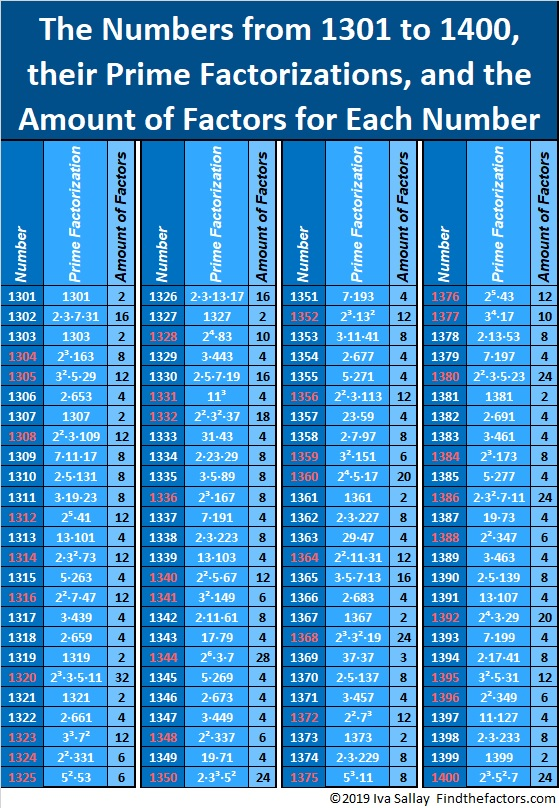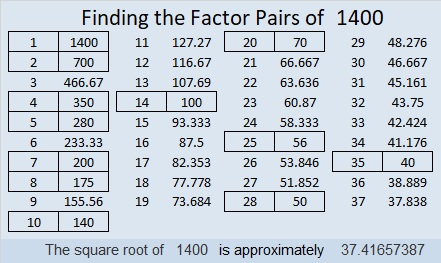# Prime Factorization of the Hundred Numbers up to 1400

Almost one-third of the numbers from 1301 to 1400 have 4 factors. Only 1/5 of the numbers have 8 factors.Since 1/3 is significantly bigger than 1/5, the amount of factors for these numbers wouldn’t make a very exciting horse race. Here is the breakdown:

• 11 numbers had 2 factors
• 1 number had 3 factors
• 32 numbers had 4 factors
• 7 numbers had 6 factors
• 20 numbers had 8 factors
• 2 numbers had 10 factors
• 13 numbers had 12 factors
• 4 numbers had 16 factors
• 1 number had 18 factors
• 2 numbers had 20 factors
• 5 numbers had 24 factors
• 1 number had 28 factors
• 1 number had 32 factors

The rosy looking numbers have square roots that can be simplified, and that is only 37% of the numbers listed.

You may not expect it, but 1400 is one of the numbers with 24 factors. Let me tell you a little bit about 1400 and why it has so many factors:

• 1400 is a composite number.
• Prime factorization: 1400 = 2 × 2 × 2 × 5 × 5 × 7, which can be written 1400 = 2³ × 5² × 7
• 1400 has at least one exponent greater than 1 in its prime factorization so √1400 can be simplified. Taking the factor pair from the factor pair table below with the largest square number factor, we get √1400 = (√100)(√14) = 10√14
• The exponents in the prime factorization are 3, 2, and 1. Adding one to each exponent and multiplying we get (3 + 1)(2 + 1)(1 + 1) = 4 × 3 × 2 = 24. Therefore 1400 has exactly 24 factors.
• The factors of 1400 are outlined with their factor pair partners in the graphic below.1400 is the hypotenuse of TWO Pythagorean triples:
392-1344-1400 which is (7-24-25) times 56
840-1120-1400 which is (3-4-5) times 280

This site uses Akismet to reduce spam. Learn how your comment data is processed.